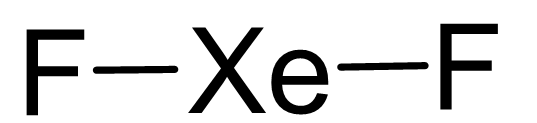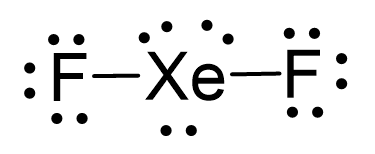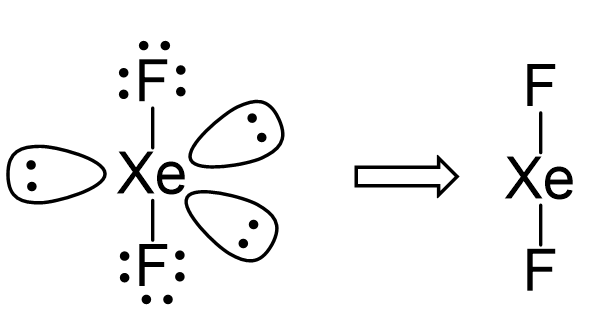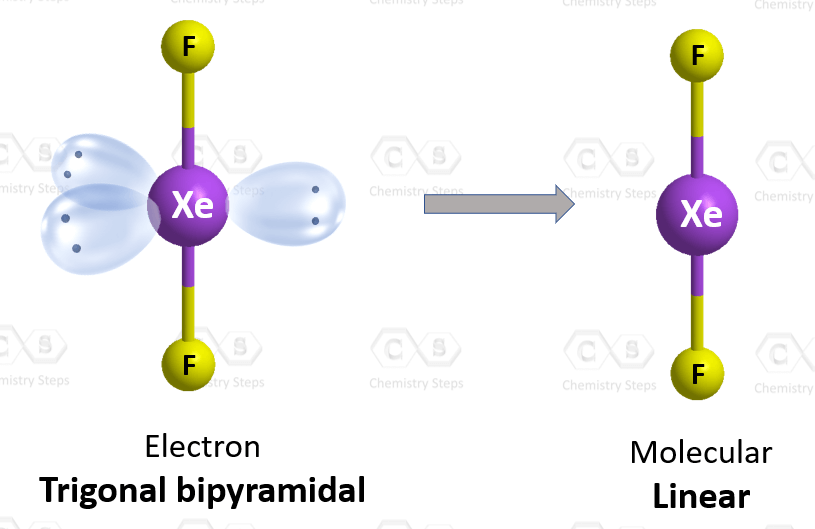## Examples

Xe is the central atom, so we can draw a preliminary skeletal structure:There are 2×7 + 8 = 22 electrons and 4 are taken to make 2 covalent bonds. Each fluorine takes 3 lone pairs, so there are 22 – (4+2×6) = 6 electrons left which go to Xe as 3 lone pairs:There are 2 atoms and 3 lone pairs on the central atom, so the steric number is 5, and therefore, the electron geometry is trigonal bipyramidal and the molecular geometry is liner:There are 5 units (atoms and lone pairs) on the central atom, and to accommodate them, it needs 5 orbitals which is achieved through sp3d hybridization.

More practice examples on geometry and hybridization in this multiple-choice quiz:

Check Also